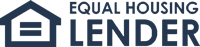# Interest Rate Pricing Explained

## How does Interest Rate Pricing Work?

Borrowers often ask the question, “What is the mortgage interest rate at today?”  The correct answer to this question is that there is not a single answer to this question.  Rates are dependent on many variables such as FICO credit score, Loan-to-Value (LTV) ratio, loan type, etc.  And most importantly, rates depend on the upfront cost or credit associated with that rate.  The Borrower ultimately chooses which rate is right for them based on the cost or credit for that rate.

## Rates are quoted in 1/8th of a Percentage

First, understand that mortgage interest rates are expressed using fractions of 1/8th of a percent sometimes written as fractions, but more often written as decimals.  So 4-1/8% will be express as the decimal 4.125% or 5-5/8% will be the decimal 5.625%.  If the current interest rate for a particular loan scenario is 4.500% and rates rise by 1/8%, the new rate would be 4.625%.  If rates move down by an eighth, the new rate would be 4.375%.

## The Interest Rate you choose will have an associated COST or CREDIT

Next, understand that every interest rate has an associated cost or credit that comes with that rate.  This means that the borrower can choose the rate they prefer based on how much they would like to pay or how much of a credit they would like to receive for the associated rate.  The higher the rate, the more credit the borrower receives.  The lower the rate, the more the borrower must pay up-front.

The chart above illustrates the interest rate pricing at a particular snapshot in time for a 30-year, fixed rate loan with a principal balance of \$300,000.  The cost/credit of each interest rate is represented by the downward sloping black line.  If the borrower chooses the 5.125% (five and an eighth) rate, the borrower will receive a \$2,800 credit toward closing costs and the borrower’s monthly principal and interest (P&I) payment will be \$1,633 per month.  If the borrower chooses the 4.750% (four and three quarters) rate, the borrower will be required to pay a cost of \$2,200 at closing and will have a monthly P&I payment of \$1,564 per month.

## Understanding “Par”

The Par Rate is the interest rate at which there is zero cost or credit associated with the rate.  It is uncommon however, for Par to fall on a particular interest rate.  Most often, Par is between two rates.  The interest rate just above Par will give a small credit and the rate just below Par will create a small cost to the borrower.

When market forces move interest rates higher or lower, what is actually happening is the Par line is moving left if rates are dropping or right if rates are rising.  Higher rates causes the borrower to pay more for the same rate.  Falling rates results in the borrower paying less for the same rate.

Since Rates are always moving and sometimes only fractions of a percentage at a time, there is not typically a specific Par Rate.  Rather, Par will rest between two interest levels, one with a slight cost and one with a slight credit.

In the end, the borrower chooses the rate based on the amount they want to spend.  If the Borrower chooses a rate below Par, the Borrower will pay a fee for that rate.  If the Borrower chooses a rate above Par, the borrower will receive a credit toward closing costs in return for accepting a higher interest rate.

## Which is the Best Choice?

In our next article, we will discuss when it is better to choose a higher rate and receive a credit, or choose the lower rate and pay the fee.

# Scott HansonDRE #01857721 NMLS #1822072
Subscribe
Stay up to date with our latest real estate insights